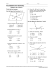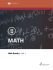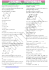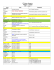Year 11 Plane Geometry Worksheet

Transcription

Year 11 Plane Geometry Worksheet
Year 11 Plane Geometry Worksheet
1.
In fig. below, S and T are points on the sides PQ and PR, respectively of ΔPQR, such that
PT = 2 cm, TR = 4 cm and ST is parallel to QR. Prove that the ratio of the areas of ΔPST
and ΔPQR is 1:9.
2.
In fig. below, ΔAHK is similar to ΔABC. If AK = 10 cm, BC = 3.5 cm and HK = 7 cm. Prove
that AC = 5 cm.
3.
If all the sides of a parallelogram ABCD touch a circle, prove that the parallelogram ABCD
is a rhombus.
4.
Find the sum of all angles of a regular hexagon.
5.
Two sides of a right angle triangle are 12 and 13. What are possible the length of the third
side?
6.
If diagonal of a square room is d, what is the area of the room?
Year 11 Plane Geometry Worksheet
P a g e |1
Year 11 Plane Geometry Worksheet
7.
In figure below, ABC is a right triangle, right angled at C and D is the mid-point of BC.
Show that 4AD2 − 3AC2 - AB2 = 0
8.
The lengths of two parallel sides of a trapezium ABCD are 6 cm and 8 cm. Its area is 42 cm2.
Find its height h.
9.
In the figure above, the PQRS is a parallelogram. Find the value of y – x =
Year 11 Plane Geometry Worksheet
P a g e |2
Year 11 Plane Geometry Worksheet
10.
Find the area of shaded region in figure below, if AC = 24 cm, BC = 10 cm and O is the
centre of the circle. [Use π = 3.14]
Year 11 Plane Geometry Worksheet
P a g e |3

Page 1 Proving a triangle is a right triangle Method 1: Show two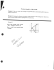Document 6575825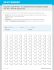Geometry Chapter 6 REVIEW Problems 3/4/2015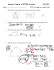Africa Political Map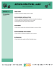Geometry Unit 1 Review Worksheet Please put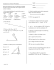Informational Interview Worksheet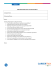BLRA eLearning Day – February 13, 2015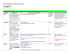Math Analysis Honors – Worksheet 44 Multivariate Linear Systems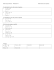Worksheets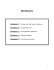A report is a description of an event or situation Clear Concise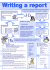Chapter One Review (Purple review worksheet)